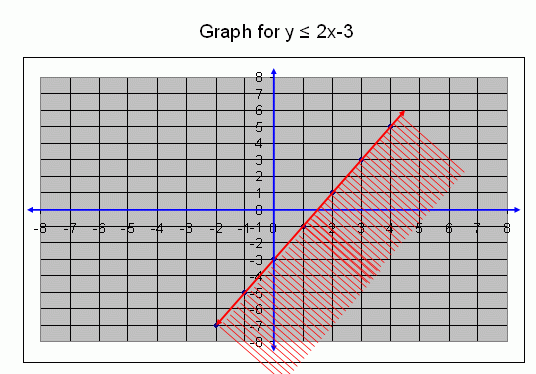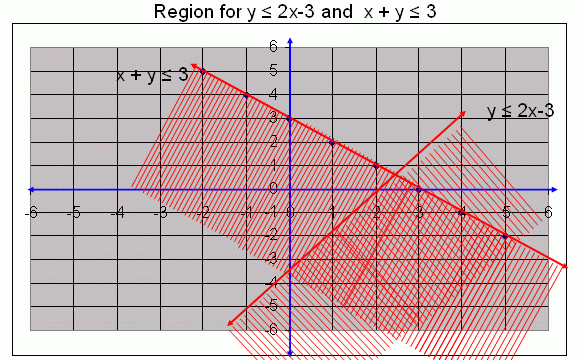Email us to get an instant 20% discount on highly effective K-12 Math & English kwizNET Programs!

#### Online Quiz (WorksheetABCD)

Questions Per Quiz = 2 4 6 8 10

### Middle/High School Algebra, Geometry, and Statistics (AGS)2.16 Linear Inequalities: Solutions Using Graphing

Graph for x + y Ł 3 and y Ł 2x - 3

Question: Graph the solution to this system of inequalities:
x + y Ł 3
y Ł 2x - 3
Solution:
First we draw a graph for y Ł 2x - 3.
Ordered pairs for y = 2x - 3

x-2-1012
y = 2x - 3-7-5-3-11
Ordered pairs(-2,-7)(-1,-5)(0,-3)(1,-1)(2,1)Any point in the cross region where the two regions overlap will satisfy x + y Ł 3 and y Ł 2x - 3
Note: A system involving parallel lines may lack a solution because there are no overlapping regions.
Example: y = x + 2 and y = x - 1

Question 1: Graph the solution to this system of inequalities: x + 2y Ł 12 and 3x - y ł 6
Question 2: Graph the solution to this system of inequalities: x ł 2 and y Ł 4 and x - y < 6